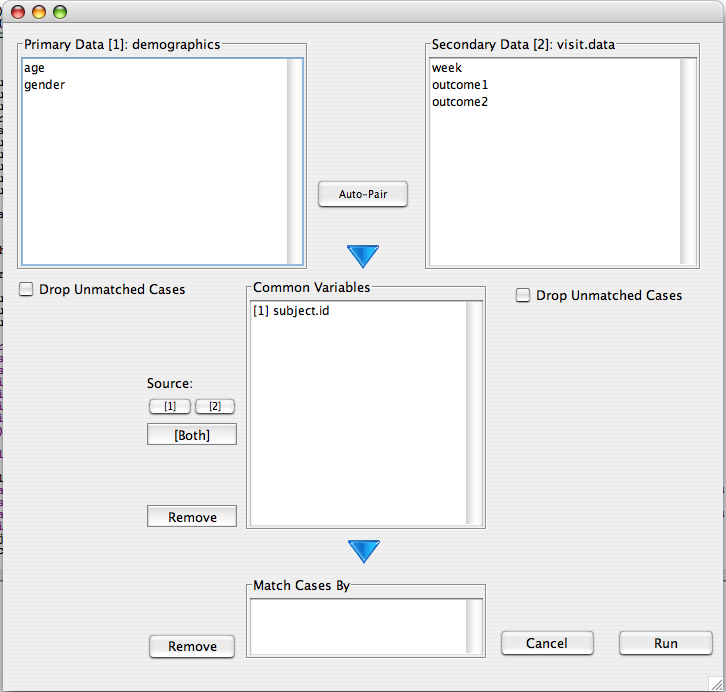## Description

Merge two data files into one based on a common identifier.

## Example Data

In this manual page two data sets will be used. the first contains demographic information (age and gender) on 10 subjects. The second contains information on two outcomes (outcome1 and outcome2) observed over three weeks. the data can be loaded with the following calls.

```demographics<-data.frame(subject.id=1:10,age=round(rnorm(10,40,10)),gender=rep(c("male","female"),5))
visit.data<-data.frame(subject.id=sort(c(1:10,1:10,1:10)),week=rep(c(1:3),10),outcome1=rnorm(30),outcome2=rnorm(30))```

## Select data

Opening the Merge Data dialog we are presented with the following dialog which we can use to merge the two data frames into a new data frame. The data frame name can be set using the box in the lower left.## Define Merge

After selecting the two data frames, all variables with a common name will be located in the common variable box. By default the resulting data will draw these variables from the primary data set (i.e. the one in the upper left). This behavior can be changed to draw a particular variable from the secondary data frame by selecting the variable and clicking the '' button. To create two different variables, one from each data frame, use the '[Both]' button.If two variables represent the same item, but are named differently in the two data frames, they can be combined by selecting both variables and clicking the down arrow. Put a unique identifier in the 'Match Cases By' list. In our example this is the `subject.id` variable. A warning will be issued if the identifiers do not uniquely secify all cases in at least one of the data frames. If no identifiers are specified, the data will be merged based on row names.

## R Code

After moving `subject.id` to the 'Match cases by' list, the following `merge` call is executed:

```demographics.temp<-demographics[setdiff(colnames(demographics),c())]
visit.data.temp<-visit.data[setdiff(colnames(visit.data),c())]
result<-merge(demographics.temp,visit.data.temp,by.x=c("subject.id"),by.y=c("subject.id"),incomparables = NA,all.x =T,all.y =T)
rm(list=c("demographics.temp","visit.data.temp"))```